# Performance Testing Web Assembly vs JavaScript

2018-05-02 Winston ChenWeb Development

### Performance Testing Web Assembly vs JavaScript

#### An experiment with some surprising results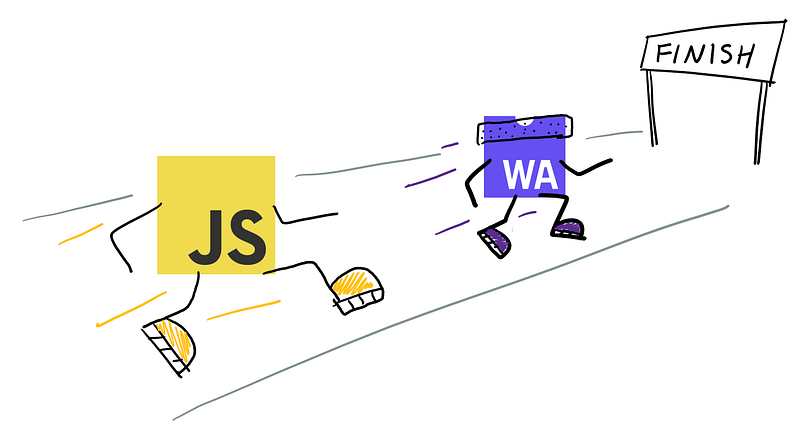Created by @diekus

The web just got a lot faster with WebAssembly, the low-level assembly-like language and compile target. The standard has reached cross-browser consensus and WebAssembly is now available in the latest Beta version of Samsung Internet (v7.2.10.12). For more background info on Web Assembly, see the article on MDN Webdocs.

WebAssembly brings near-native performance to the web. But, how much faster is WebAssembly compared to JavaScript? To find out, I created a little experiment to test how fast WebAssemby is.

If you want the tl;dr, skip ahead to the Summary section, but first a detailed description of the experiment:

### The Experiment

The experiment involves writing several implementations of matrix multiplication in JavaScript and C++. I chose matrix multiplication, because it is simple and computationally intensive (O(n³) for naive implementations). The first implementation (ijk) provides a baseline to compare other implementations. The second implementation (kji) is similar to the first implementation, but misuses temporal locality. The third implementation (bmm) takes advantage of temporal locality. Implementations two and three try to determine if a well written function in JavaScript will outperform a poorly written function in C++.

The first implementation provides a baseline for performance where we simply iterate through the rows and columns of both matrices:

// Javascript - ijk (order of the loops)
function ijk(matrixA, matrixB, matrixC, length) {
for(let i = 0; i < length; i++){
for(let j = 0; j < length; j++) {
for(let k = 0; k < length; k++) {
matrixC[i * length + j] += (matrixA[i * length + k] * matrixB[j * length + k]);
}
}
}
}

// C++ - ijk (order of the loops)
void ijk(double* matrixA, double* matrixB, double* matrixC, int length) {
for(unsigned int i = 0; i < length; i++){
for(unsigned int j = 0; j < length; j++) {
for(unsigned int k = 0; k < length; k++) {
matrixC[i * length + j] += (matrixA[i * length + k] * matrixB[j * length + k]);
}
}
}
}

The second implementation is a slower algorithm, by interchanging the outermost loop (i) with the innermost loop (k). (Notice the summation part `matrixC[i * length + j] += (matrixA[i * length + k] * matrixB[j * length + k])` is the same as above in the first implementation). This implementation effectively degrades the memory hierarchy performance by causing more cache misses:

// Javascript - kji (order of loops)
function kji(matrixA, matrixB, matrixC, length) {
for(let k = 0; k < length; k++) {
for(let j = 0; j < length; j++) {
for(let i = 0; i < length; i++){
matrixC[i * length + j] += (matrixA[i * length + k] * matrixB[j * length + k]);
}
}
}
}

// C++ - kji (order of loops)
void kji(double* matrixA, double* matrixB, double* matrixC, int length) {
for(unsigned int k = 0; k < length; k++) {
for(unsigned int j = 0; j < length; j++) {
for(unsigned int i = 0; i < length; i++){
matrixC[i * length + j] += (matrixA[i * length + k] * matrixB[j * length + k]);
}
}
}
}

The third implementation takes advantage of a technique called blocking. Blocking takes advantage of temporal locality to increase cache hits. The block size I chose for this experiment is 32, because the array sizes are divisible by 32, and I already know small block sizes work well. I also did block sizes of 8 and 16 as well.

// Javascript - bmm (blocked matrix multiplication)
function bmm(matrixA, matrixB, matrixC, length, blockSize) {
let block = blockSize * (length/blockSize);
let sum;
for(let kk = 0; kk < block; kk += blockSize) {
for(let jj = 0; jj < block; jj += blockSize) {
for(let i = 0; i < length; i++) {
for(let j = jj; j < jj + blockSize; j++) {
sum = matrixC[i * length + j];
for(let k = kk; k < kk + blockSize; k++) {
sum += matrixA[i * length + j] * matrixB[i * length + j];
}
matrixC[i * length + j] = sum;
}
}
}
}
}

// C++ - bmm (blocked matrix multiplication)
void bmm(double* matrixA, double* matrixB, double* matrixC, int length, int blockSize ) {
unsigned int block = blockSize * (length/blockSize);
double sum;

for (unsigned int kk = 0; kk < block; kk += blockSize) {
for (unsigned int jj = 0; jj < block; jj += blockSize) {
for (unsigned int i = 0; i < length; i++) {
for (unsigned int j = jj; j < jj + blockSize; j++) {
sum = matrixC[ilength+j];
for (unsigned int k = kk; k < kk + blockSize; k++) {
sum += matrixA[i\
length+k] * matrixB[klength+j];
}
matrixC[i
length+j] += sum;
}
}
}
}
}

### Results

These graphs below show the time (milliseconds) for different array sizes ranging from 64x64 to 1024x1024 for each of the above implementations (ijk, kji and bmm ).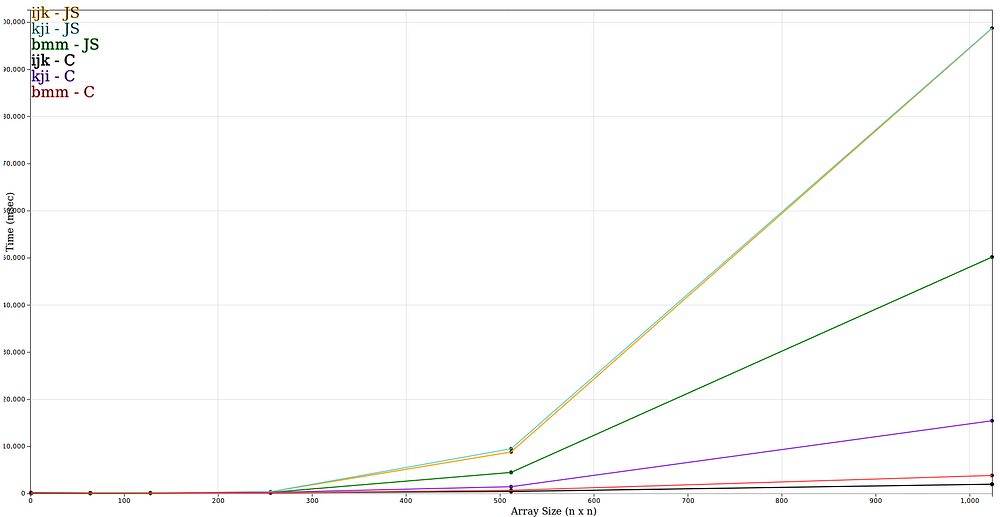msec vs array sizes 64–1024 (n x n)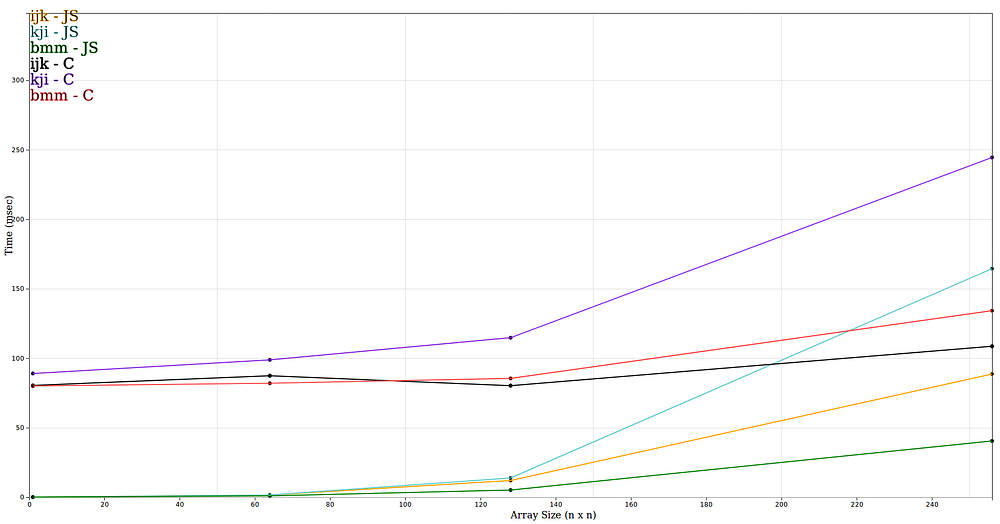msec vs array sizes 64–256 (n x n)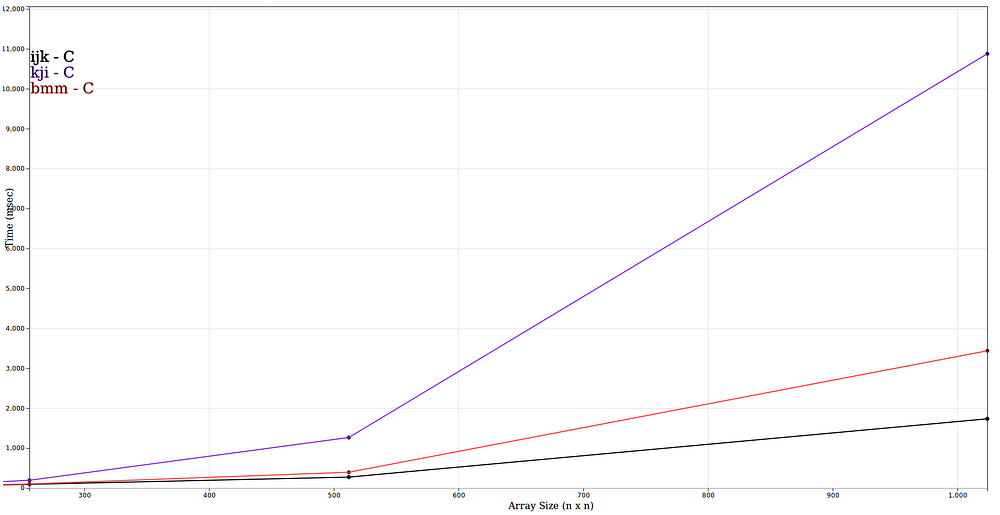msec vs array sizes 256–1024 (n x n) of the C implementations

### Analysis

Before I breakdown the results, I want to note a few things. First, I added an array size 1 to get rid of ‘noise’, where the ijk implementation in C++(black line) time for array size 1 x 1 is slower than an array size 64 x 64 according to the graph. The same would happen if I started at 64 x 64, where 64 x 64 was slower than 128 x 128. Second, the results are an average of of 25 runs. CPUs typically have multiple workloads which can add some overhead from context switching to a single run of any of the above functions. Taking the average of multiple runs helps converge to a more accurate measurement.

Overall, the WebAssembly implementations outperform the JavaScript implementations by a significant amount on the bigger matrix sizes, which is what I expected. But in the graph of array sizes 64 to 256 shows that the JavaScript implementation actually out performs the Web Assembly implementation.

There are some interesting data points. The ijk implementations for C seem to be faster for 128x128 than for the 64x64 arrays. And the blocked implementation for C has similar running times for 64x64 and 128x128 arrays. The blocked implementation in C is slower than the ijk implementation. The reason may be my compiler optimized the ijk implementation a lot better than the blocked version. The blocked version in JavaScript out performed all the other JavaScript implementations.

Below are graphs of cycle per iteration (CPI) vs. array size. CPI provides insight on how well a program utilizes the CPU. An ideal program has low CPI, where the CPU is mostly executing instructions (and not I/O bound operations). High CPI typically means the CPU spends some of the time fetching data from main memory or hard drive. CPI can be derived from the equation below:

CPI = (Time * CPU frequency) / number of instructions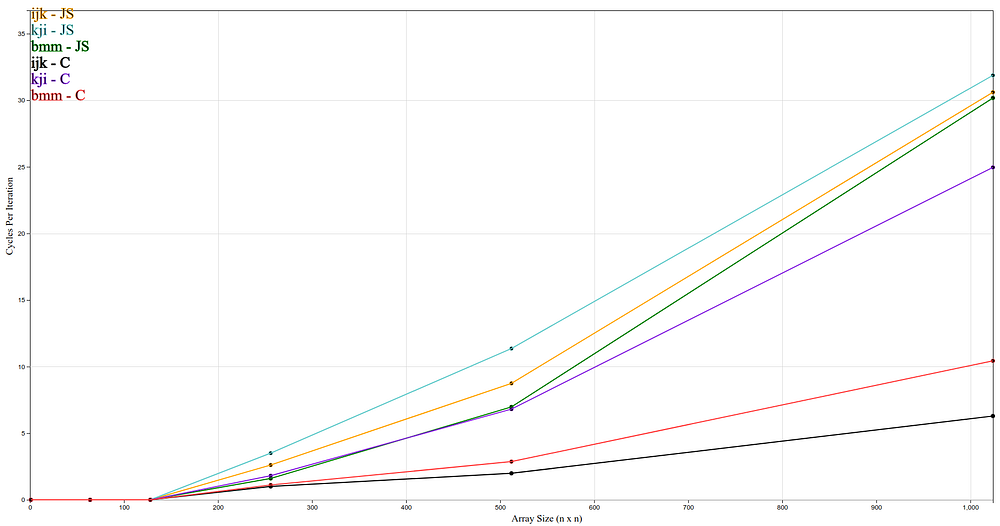Cycles per iteration vs. array size 128–1024 (n x n)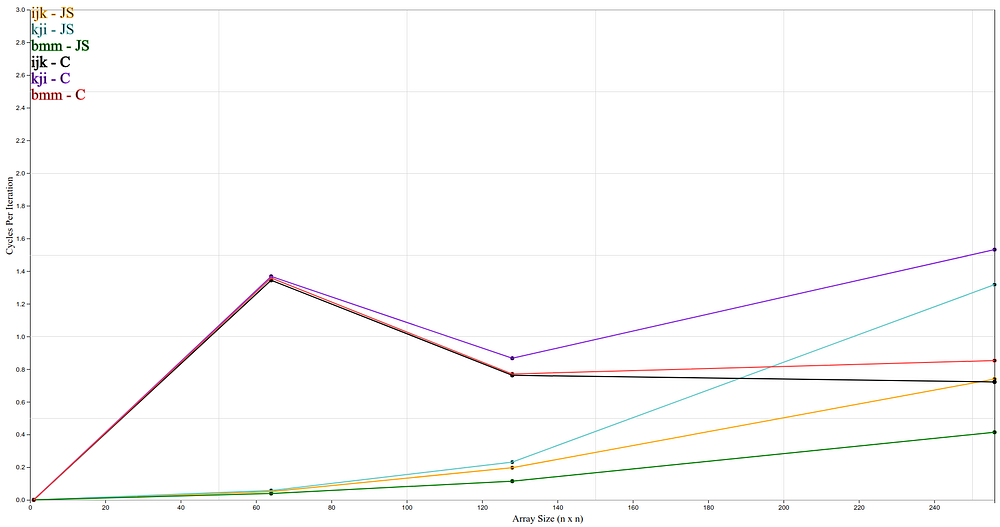Cycles per iteration vs. array size 64–256(n x n)

### Summary

JavaScript performed better than WebAssembly on smaller array sizes and WebAssembly performed better than JavaScript on larger array sizes.

From this experiment, we can expect that for most web applications, JavaScript is the best option. Web Assembly is best used for computationally intensive web applications, such as web games. However, we recommend testing to see whether a JavaScript implementation or a WebAssembly implementation provides better performance.

You can find the source code to this experiment here. You can also try this out in your browser here.

The latest Beta version of Samsung Internet (v7.2.10.12) supports Web Assembly, so feel free to test it out and tweet us (@Samsunginternet) how you utilize Web Assembly. Future stable releases of Samsung Internet will support Web Assembly too.